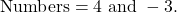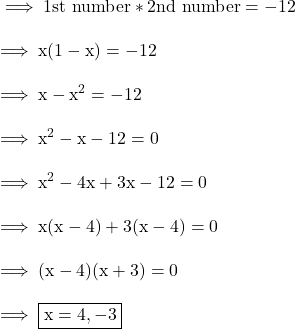## Find the TWO integers whos product is -12 and whose sum is 1 m​

Question

Find the TWO integers whos product is -12 and whose sum is 1
m​

in progress 0
2 months 2021-08-02T09:42:07+00:00 2 Answers 2 views 0

-3,4 are the TWO integers whos product is -12 and whose sum is 1.

Step-by-step explanation:

-3×4=12

-3+4=1Step-by-step explanation:

Given :-

The sum of two numbers is 1 .

The product of the nos . is 12 .

And we need to find out the numbers. So let us take ,

First number be x

Second number be 1-x .

According to first condition :-Hence the numbers are 4 and -3.